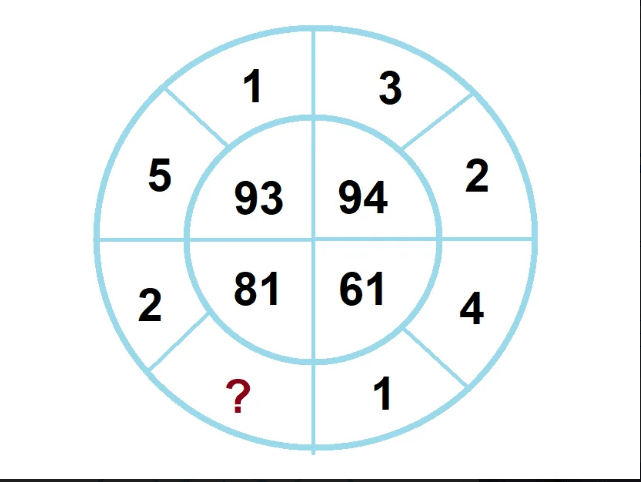# Math puzzle «for 1 minute»NewsThis puzzle conquered the Internet. If you have a free moment and want to train your brain, then consider this math puzzle.

As you know, math puzzles help develop patience and logical thinking, and this is a great opportunity to once again see how smart you are.
And the task is this: you need to find a pattern in these numbers and determine which number is missing.
To solve this problem, you will need to determine the logic by which the numbers are arranged. The answer, in fact, lies right on the surface, try to look at this circle through the eyes of a child!

Explanation will be below.Don't rush to look at the answer, you will be able to come up with the right solution yourself!
Guessed it? Well done!

Solution:

And the solution is this: the sum of the numbers standing separately in each quadrant, is equal to the difference of numbers standing together in the same quadrant. Now everything will become clear:

Upper circle on the right:
3+2=5
9−4=5
Lower circle on the right:
1+4=5
6−1=5
Upper circle left:
5+1=6
9−3=6
And bottom circle left:
8−1=7
Accordingly, the sum is 2+?=7. It turns out 2+5=7
Agree that this puzzle is a great opportunity to stir the mind!
What is your IQ: a short IQ test, see here and another one here

Оцените статью